• ## python 常用运算符

万次阅读 2016-07-23 00:47:38
和其他大多数的语言一样，python 也有 +（加）、-（减）、*（乘）、/（除）、%（取余）这 5 个运算符，除此之外还有两个特殊的运算符，分别是 //（整除）和 **（幂运算符，或叫乘方运算符）。 这里，+、-、* 的功能...
和其他大多数的语言一样，python 中常用的操作符也有算术操作符、比较操作符、逻辑操作符，但是又有一些差别，下面详细介绍。
1. 算术运算符
和其他大多数的语言一样，python 也有 +（加）、-（减）、*（乘）、/（除）、%（取余）这 5 个运算符，除此之外还有两个特殊的运算符，分别是 //（整除）和 **（幂运算符，或叫乘方运算符）。
这里，+、-、* 的功能和计算器相同，不再赘述了。
% 运算符对浮点数也有效，例如：
>>> 3.75 % 0.5
0.25
>>> 3.75 % 2
1.75
>>>

** 运算符是进行幂（乘方）运算的，例如：
>>> 3**2
9
>>> 2**3
8
>>>
需要注意的是 ** 运算符和正负号（+、-）之间的优先级关系，** 比其左侧的正负号优先级高，比其右侧的正负号优先级低。或者直接就可以认为 ** 运算符比正负号优先级高，因为正负号在其右侧时，两个符号在一起，总不能把正负号去掉吧。例如：

>>> -3**2
-9
>>> 3**-2
0.1111111111111111
>>>

需要强调和区别的是 / 和 // 这两个除运算符。python 2.7 中，/ 对于两个整数相除来说是整除，计算结果只留下整数部分，而小数部分没有了，例如：
>>> 3/2
1
>>>
如果想要执行普通的除法，即实现保留计算结果的小数部分，可以用浮点数进行运算，例如：

>>> 3.0/2
1.5
>>> 3/2.0
1.5
>>> 3/2.
1.5
>>> 3.0/2.0
1.5
>>>
而在 python 3 中，/ 有所改变，不管是整数除法还是浮点数除法，执行的都是浮点数除法，即可以保留计算结果的小数部分。

同时，在 python 2 中实现保留计算结果的小数部分的另一个方法是，在程序前加上一句 from __feture__ import division 并执行该语句（语句中的 __ 为两个下划线），例如：

>>> from __future__ import division
>>> 3/2
1.5
>>> 5/4
1.25
>>>
此时的除法运算和 python 3 中除法运算已经是一致的了。

在 python 中还提供了 // 这个整除运算符，它做的是整除，而且对浮点数执行的也是整除，例如：

>>> 3//2
1
>>> 3.0//2.0
1.0
>>> 5//2.0
2.0
>>>

2. 比较运算符
和其他大多数的语言一样，python 的比较运算符包括 <、<=、>、>=、==、!=。比较运算符根据表达式的值的真假返回布尔值 True 或 False。例如：

>>> 3 < 4
True
>>> 3 > 4
False
>>> 3 == 4
False
>>> 3 != 4
True
>>>

3. 逻辑运算符
逻辑运算符就是我们常说的与、或、非，在 python 里分别表示为 and、or、not。
使用逻辑运算符可以将任意的表达式连接在一起，并得到一个布尔值。例如：
>>> 3 < 4 and 3 > 4
False
>>> 3 < 4 or 3 == 4
True
>>> not 3 < 4
False
>>>
在使用逻辑运算符时，最需要注意的一点是短路逻辑（或叫做惰性求值），它表达的意思是：逻辑运算是自左向右进行的，如果左边已经决定结果，就不会再去做右边的计算。具体的表示如下：

对于 x and y，如果 x 为假，则立刻返回 x 的值，而不用去执行 y；如果 x 为真，则返回 y 的值对于 x or y，如果 x 为真，则立刻返回 x 的值，而不用去执行 y；如果 x 为假，则返回 y 的值
例如：

>>> a
Traceback (most recent call last):
File "<pyshell#21>", line 1, in <module>
a
NameError: name 'a' is not defined

>>> 0 and a
0

>>> 0 or a
Traceback (most recent call last):
File "<pyshell#23>", line 1, in <module>
0 or a
NameError: name 'a' is not defined

>>> 6 and a
Traceback (most recent call last):
File "<pyshell#24>", line 1, in <module>
6 and a
NameError: name 'a' is not defined

>>> 6 or a
6
>>>

由于我们提前没有定义变量 a，所以执行 a 时是会报错的。

对于 0 and a，由于先判断 0 为假（程序语言中一般 0 都表示假，非 0 代表真），此时已经能够决定整个结果是假，所以会直接返回 0 而不去执行 a，因而不会报错。对于 0 or a，先判断 0 为假，此时并不能决定整个结果是真是假，所以会继续去执行 a，因而就会报错。对于 6 and a，先判断 6 为真，此时并不能决定整个结果是真是假，所以会继续去执行 a，因而就会报错。对于 6 or 啊，由于先判断 6 为真，此时已经能够决定整个结果是真，所以会直接返回 6 而不去执行 a，因而不会报错。

总结：运算符的优先级
下面我们再来总结运算符的优先级高低，见下图：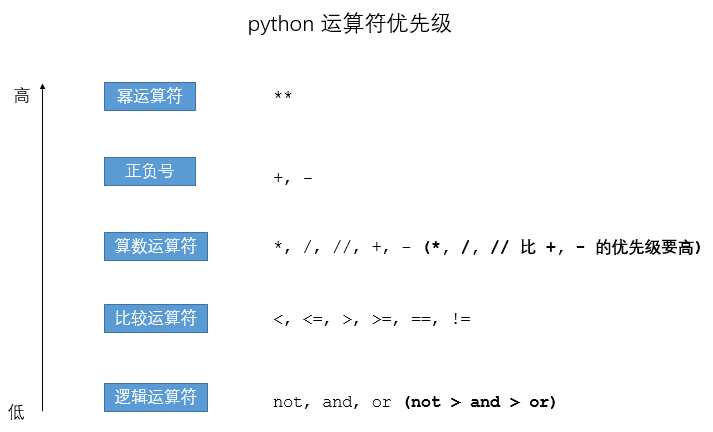展开全文运算符 短路逻辑
• 本文介绍使用Python来做数学计算，在学习和工作中，经常会遇到一些数学计算的问题。一般人会使用计算器软件，不得不说，计算器太难用了。专业人士可能会使用更强大的工具，如Matlab，但这种重量级工具有时可能并不...
原文链接: http://zh.5long.me/2015/python-math-1/

摘要（Abstract）

本文介绍使用Python来做数学计算，在学习和工作中，经常会遇到一些数学计算的问题。一般人会使用计算器软件，不得不说，计算器太难用了。专业人士可能会使用更强大的工具，如Matlab，但这种重量级工具有时可能并不适用。本文打算使用一个轻量级的工具Python来做计算。准确来说Python并不是一个数学工具，而是一种编程语言。Python提供了很多数学库，利用Python和这些库可以做很多数学计算。

本文不是编程语言的教程，更像是一个工具的使用教程，阅读本文不需要有程序设计基础，当然，需要一点数学基础（比如加减乘除）。本文适合任何想找一个计算工具的人学习和参考。

本文将以实例讲解各种用法。

安装Python（Installation）

提示1：Mac OS和大部分版本Linux系统自带Python运行环境，可以不用安装。当然，也可升级成最新版本。Windows需要自行安装。
提示2：也可以安装Sublime Text编辑器，再安装Sublime REPL插件。本人现在使用这种方案，写Python程序非常方便。

提示3：搜狗输入法用户注意，搜狗输入法在IDLE下有点小问题（不能退格），切换到英文输入法即可。

Python 2 和Python 3的注意事项

print的语法。python 3的用法为print("hello world!")，python 2的用法为print "hello world!"或者print("hello world!")。

基本运算

加法

>>> 1 +  2      //直接输入，回车直接输出结果
3
>>> sum = 1 + 2 //计算结果保存在sum中
>>> print(sum)      //输出sum
3
>>> a = 1       //变量
>>> b = 2
>>> sum = a + b     //变量相加
>>> print(sum)
312345678910

减法

>>> a = 1
>>> b = 2
>>> 2 - 1
1
>>> a - b
-1
>>> b - a
112345678

乘法

>>> 1 * 2
2
>>> 1.5 * 3
4.5
>>> a * b
2
>>> 1234567

除法

传统的除法。有编程经验的人一定知道，整型除法的结果也是整型（地板除，舍弃小数部分）。如果有一个数是浮点型，则结果是浮点型（真正的除法，保留小数）。

>>> 1 / 3           //整型除法，商为0，舍弃小数部分
0
>>> 5 / 2
2
>>> 1.0 / 3         //浮点数除法，保留小数
0.3333333333333333
>>> 5.0 / 2.0
2.512345678

真正的除法。在未来的Python版本中，不管是整型还是浮点型除法，结果总是真实地商（保留小数部分），现阶段可以通过执行from __future__ import division指令做到这一点。

>>> from __future__ import division
>>> 1 / 3
0.3333333333333333
>>> 5 / 2
2.5
>>> 1.0 / 3
0.3333333333333333
>>> 5.0 / 2.0
2.5123456789

地板除。Python 2.2新加了一个操作符//，//除法不管操作数为何种数值类型，总是舍弃小数部分。

>>> 1 // 3
0
>>> 5 // 2
2
>>> 1.0 // 3
0.0
>>> 5.0 // 2.0
2.012345678

取余数

>>> 1 % 3
1
>>> 5 % 2
1
>>> 5.0 % 2.0
1.0123456

幂运算

Python有幂运算符**。

>>> 2 ** 3
8
>>> 2.5 ** 5
97.65625
>>> 4 ** -1
0.25123456

代码中分别计算23、2.55、4-1。

复数

复数的表示。复数的表示如下：

>>> aComplex = 1 + 2j       //申明一个复数
>>> aComplex
(1+2j)
>>> aComplex.real           //复数实部
1.0
>>> aComplex.imag           //复数虚部
2.0
>>> aComplex.conjugate()        //共轭复数
(1-2j)
>>> 12345678910

复数的运算。复数的运算与实数一致。

>>> c = 1 + 2j
>>> d = 2 - 1j
>>> c + d
(3+1j)
>>> c - d
(-1+3j)
>>> c * d
(4+3j)
>>> c / d
1j
>>> c / 2
(0.5+1j)
>>> c * 2
(2+4j)
>>> c ** 2
(-3+4j)12345678910111213141516

math标准库

Python有一个标准库math专门用来做数学运算的。详细介绍可参考Python的官方文档。要使用math库，先要import这个库。

>>> import math1

一下的例子假设已经执行了import math。

两个常数

>>> math.pi             //圆周率pi
3.141592653589793
>>> math.e
2.718281828459045       //自然常数e1234

数值计算

math.ceil(x)。向上取整，返回最小的大于或等于x的整数。

>>> math.ceil(2)
2.0
>>> math.ceil(2.2)
3.0
>>> math.ceil(2.9)
3.0
>>> math.ceil(3.0)
3.012345678

math.floor(x)。向下取整，返回最大的小于或等于x的整数。

>>> math.floor(2)
2.0
>>> math.floor(2.2)
2.0
>>> math.floor(2.9)
2.0
>>> math.floor(3.0)
3.012345678

math.fabs(x)。取x得绝对值。

>>> math.fabs(1.0)
1.0
>>> math.fabs(-1.0)
1.01234

math.factorial(x)。求x的阶乘，x必须为整数，否则出现错误。

>>> math.factorial(5)
120
>>> math.factorial(4)
24
>>> math.factorial(2.1)     //执行错误
Traceback (most recent call last):
File "<stdin>", line 1, in <module>
ValueError: factorial() only accepts integral values
>>> 123456789

幂和对数函数（Power and logarithmic functions）

math.exp(x)。返回e ** x。

>>> math.exp(2)
7.38905609893065
>>> math.e ** 2
7.3890560989306495  //请忽略后面的不一致，计算机浮点数本身的问题1234

math.log(x [,base])。求以base为底的对数。

>>> math.log(math.e)        //值传一个参数，默认以math.e为底
1.0
>>> math.log(math.e ** 2)
2.0
>>> math.log(8, 2)      //两个参数，2为底
3.0
>>> math.log(100, 10)   //两个参数，10为底s
2.012345678

math.pow(x, y)。幂运算，计算xy，相当于x ** y。

>>> math.pow(2, 3)
8.0
>>> 2 ** 3
81234

math.sqrt(x)**。求x的平方根。

>>> math.sqrt(4)
2.0
>>> math.sqrt(9.0)
3.01234

开根号。Python的math库中只有开平方根，没有立方根和n次方根，不过可以利用math.pow或者**，只需把根号变成分数。

>>> math.pow(4, 1.0 / 2)        //平方根，相当于math.sqrt(4)
2.0
>>> 4 ** (1.0 / 2)          //平方根，相当于math.sqrt(4)
2.0
>>> 8 ** (1.0 / 3)          //立方根
2.0
>>> 1024 ** (1.0 / 10)          //10次方根
2.012345678

三角函数（Trigonometric functions）

方法名
实现功能

math.degrees(x)
把弧度x转换成角度

把角度x转换成弧度

>>> math.degrees(math.pi)
180.0
3.1415926535897931234

math.cos(x)。不解释。
math.sin(x)。不解释。
math.tan(x)。不解释。
math.acos(x)。反余弦函数。
math.asin(x)。反正弦函数。
math.atan(x)。反正切函数。
math.hypot(x，y)。欧式范数（Euclidean norm）。相当sqrt(x*x + y*y)。

>>> math.cos(math.pi)
-1.0
>>> math.sin(math.pi / 2)
1.0
>>> math.tan(math.pi / 4)
0.9999999999999999      //结果为1，计算机浮点数固有缺陷
>>> math.tan(math.pi / 4)
0.9999999999999999
>>> math.acos(-1.0)
3.141592653589793
>>> math.asin(1.0)
1.5707963267948966
>>> math.atan(1)
0.7853981633974483
>>> math.pi / 4
0.7853981633974483
>>> math.hypot(3, 4)
5.0123456789101112131415161718

双曲函数（Hyperbolic functions）

双曲函数和三角函数类似。

math.cosh(x)。求x的双曲余弦函数。
math.sinh(x)。求x的双曲正弦函数。
math.tanh(x)。求x的双曲正切函数。
math.acosh(x)。求x的反双曲余弦。
math.asinh(x)。求x的反双曲正弦。
math.atanh(x)。求x的反双曲正切。

>>> math.cosh(1)
1.5430806348152437
>>> math.sinh(1)
1.1752011936438014
>>> math.tanh(1)
0.7615941559557649
>>> math.acosh(1.5430806348152437)
1.0
>>> math.asinh(1.1752011936438014)
1.0
>>> math.atanh(0.7615941559557649)
0.9999999999999999          //结果为1，计算机浮点数固有缺陷123456789101112

一个复杂的例子

举一个复杂的例子，计算下面的公式：

>>> - (4 ** (1.0 / 3)) / 4 * math.log(2 ** (1.0 / 3) - 1) - (math.sqrt(3) * (4 ** (1.0 / 3))) / 6 * math.atan(math.sqrt(3) / (1 + 2 * (2 * (1.0 / 3))))
0.2420914086273389712

坑

在前面的例子可能已经注意到了，计算机表示浮点数是不准确的，比如1.0成了0.9999999999999999。这是计算机浮点数表示法的固有缺陷。比如会出现如下结果：

>>> 0.05 + 0.01
0.060000000000000005
>>> 1.0 - 0.12
0.88
>>> 1.0 - 0.32
0.6799999999999999
>>> 1.0 - 0.42
0.5800000000000001
>>> 4.015 * 100
401.49999999999994
>>> 123.3 / 100
1.2329999999999999123456789101112

浮点数的缺陷不仅仅在Python在存在，在任何语言都存在，它是浮点数表示法本身的缺陷。对于不是非常严格的计算，这种缺陷是可以接受的，而对于过于严格的计算，那么应该用整型数计算（整型计算是精确的）。比如在保留两位小数的计算中，可以把原始数乘以100取整数再计算，约定最后两位为小数即可。

结语

本文介绍了Python标准库的数学计算方法，通过使用Python标准数学库，可以应付一些日常的基本计算（比如我就很少使用计算器了，一般的计算都用Python解决）。当然，数学是如此的丰富，仅有标准库是远远不够的。将来如果有需要的话，我会研究下使用其它的数学库来进行更复杂的计算以及数学图像的绘制。比如NumPy、SciPy、Matplotlib、SymPy等。

函数定义

在python中函数的定义以及调用如下代码所示：

def test(x):
y = x+1
return y

result = test(2)
print(result)

多个返回值的情况

如果在函数中return多个值，会将那多个值打包成一个元组传出，如下代码所示def test(x):
y1 = x+1
y2 = x+2
return y1,y2

result = test(2)
print(result) #打印结果为(3, 4)使用关键字参数的情况

使用关键字参数，则传参的位置可以不固定，但是个数还是要相匹配，此外在调用的时候关键字参数一定要在普通参数的右边，如下代码所示

def test(x,y,z):
res = x - y - z
return res

result = test(y=1,x=5,z=2)
print(result) #打印结果为2

使用默认参数的情况

使用默认参数，传参的个数可以不匹配，如果默认参数的位置没有传，则使用默认值，这个是在定义的时候定义的，定义默认参数要写在最右边，如下代码所示def test(name,sex = "man"):
print(name)
print(sex)

test("CodeScrew")
#打印结果为CodeScrew  man
test("CodeScrew","women")
#打印结果为CodeScrew  women使用参数组的情况

参数组中指的是可以传任意参数，一般**对应字典，*对应列表，如下代码所示

传入列表
def test(name,*args):
print(name)
print(args)

test("CodeScrew",*[1,2,3,4])
#打印结果为CodeScrew   (1, 2, 3, 4)

传入字典def test(name,**kwargs):
print(name)
print(kwargs)

test("CodeScrew",x=1,y=2)
#打印结果为CodeScrew   {'x': 1, 'y': 2}
test("CodeScrew",**{"x":1,"y":2})
#打印结果为CodeScrew   {'x': 1, 'y': 2}def test(x,y,z):
res = x - y - z
return res

result = test(y=1,x=5,z=2)
print(result) #打印结果为2

方法一: 使用内置模块

>>> import math >>> math.pow(12, 2)     # 求平方144.0 >>> math.sqrt(144)      # 求平方根12.0 >>>

方法二: 使用表达式

>>> 12 ** 2             # 求平方144 >>> 144 ** 0.5          # 求平方根12.0 >>>

方法三: 使用内置函数

>>> pow(12, 2)          # 求平方144 >>> pow(144, .5)        # 求平方根12.0


展开全文• 逻辑运算 指数运算 整除 所以，我们来做个运算吧~ 用raw_input()可以从键盘上读取输入，raw_input()中的字符串会在屏幕上面打印出来 用int()转只因为Python默认都是以str(字符串)类型来接收输入的...
逻辑运算指数运算整除所以，我们来做个运算吧~用raw_input()可以从键盘上读取输入，raw_input()中的字符串会在屏幕上面打印出来
用int()转只因为Python默认都是以str(字符串)类型来接收输入的，所以想要加减乘除计算就只好强转喽~
让我们来跑一下：字符串运算也可以哦：Python字符串默认是从左向右取得哦，所以要想倒着取值还是有点考量的：其中，最后一句 a[4:1:-1] 里的 -1 可以看作是取值的步数
转载于:https://www.cnblogs.com/fallenmoon/p/6938373.html
展开全文• ## Python3运算符号

万次阅读 多人点赞 2019-01-23 22:50:15
本章节主要说明Python的运算符。举个简单的例子 4 +5 = 9 。 例子中，4 和 5 被称为操作数，&quot;+&quot; 称为运算符。 Python语言支持类型的运算符: 算术运算符 比较（关系）运算符 赋值运算符 逻辑...
什么事运算符？
本章节主要说明Python的运算符。举个简单的例子 4 +5 = 9 。 例子中，4 和 5 被称为操作数，"+" 称为运算符。

Python语言支持类型的运算符:
算术运算符
比较（关系）运算符
赋值运算符
逻辑运算符
位运算符
成员运算符
身份运算符
运算符优先级

Python算术运算符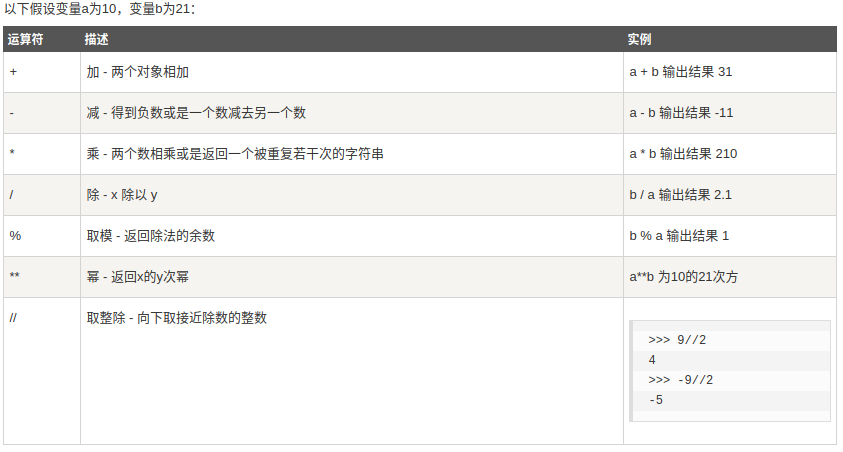实例：
#!/usr/bin/python3

a = 21
b = 10
c = 0

c = a + b
print ("1 - c 的值为：", c)

c = a - b
print ("2 - c 的值为：", c)

c = a * b
print ("3 - c 的值为：", c)

c = a / b
print ("4 - c 的值为：", c)

c = a % b
print ("5 - c 的值为：", c)

# 修改变量 a 、b 、c
a = 2
b = 3
c = a**b
print ("6 - c 的值为：", c)

a = 10
b = 5
c = a//b
print ("7 - c 的值为：", c)

输出结果：
1 - c 的值为： 31
2 - c 的值为： 11
3 - c 的值为： 210
4 - c 的值为： 2.1
5 - c 的值为： 1
6 - c 的值为： 8
7 - c 的值为： 2

Python比较运算符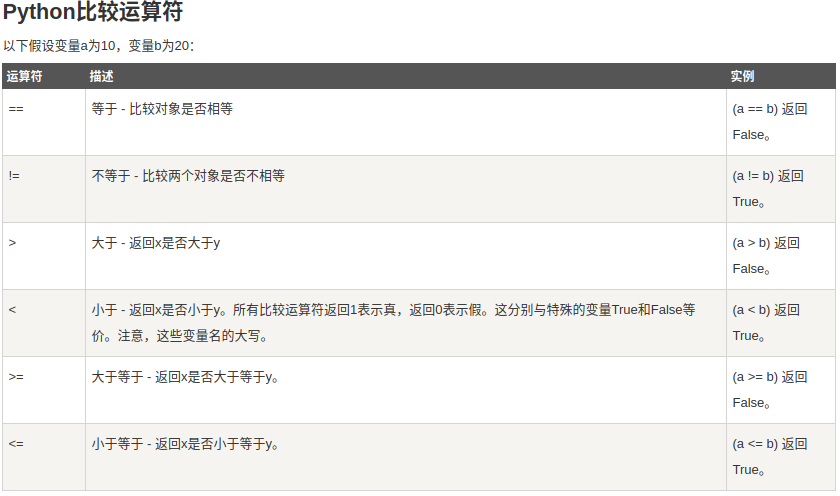实例：
#!/usr/bin/python3

a = 21
b = 10
c = 0

if ( a == b ):
print ("1 - a 等于 b")
else:
print ("1 - a 不等于 b")

if ( a != b ):
print ("2 - a 不等于 b")
else:
print ("2 - a 等于 b")

if ( a < b ):
print ("3 - a 小于 b")
else:
print ("3 - a 大于等于 b")

if ( a > b ):
print ("4 - a 大于 b")
else:
print ("4 - a 小于等于 b")

# 修改变量 a 和 b 的值
a = 5;
b = 20;
if ( a <= b ):
print ("5 - a 小于等于 b")
else:
print ("5 - a 大于  b")

if ( b >= a ):
print ("6 - b 大于等于 a")
else:
print ("6 - b 小于 a")

输出结果：
1 - a 不等于 b
2 - a 不等于 b
3 - a 大于等于 b
4 - a 大于 b
5 - a 小于等于 b
6 - b 大于等于 a

Python赋值运算符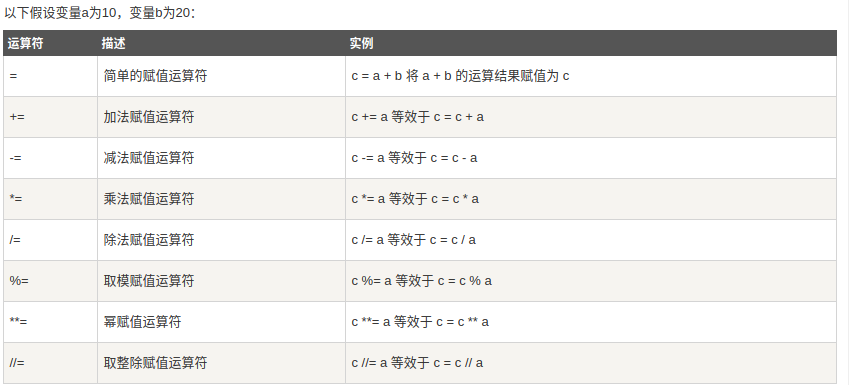实例：
#!/usr/bin/python3

a = 21
b = 10
c = 0

c = a + b
print ("1 - c 的值为：", c)

c += a
print ("2 - c 的值为：", c)

c *= a
print ("3 - c 的值为：", c)

c /= a
print ("4 - c 的值为：", c)

c = 2
c %= a
print ("5 - c 的值为：", c)

c **= a
print ("6 - c 的值为：", c)

c //= a
print ("7 - c 的值为：", c)

输出结果：
1 - c 的值为： 31
2 - c 的值为： 52
3 - c 的值为： 1092
4 - c 的值为： 52.0
5 - c 的值为： 2
6 - c 的值为： 2097152
7 - c 的值为： 99864

Python位运算符
按位运算符是把数字看作二进制来进行计算的。Python中的按位运算法则如下：
下表中变量 a 为 60，b 为 13二进制格式如下：
a = 0011 1100
b = 0000 1101

a&b = 0000 1100
a|b = 0011 1101
a^b = 0011 0001
~a  = 1100 0011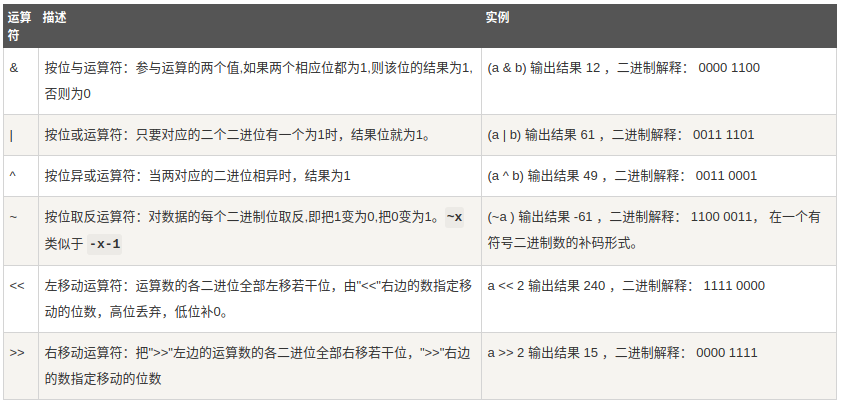实例：
#!/usr/bin/python3

a = 60            # 60 = 0011 1100
b = 13            # 13 = 0000 1101
c = 0

c = a & b;        # 12 = 0000 1100
print ("1 - c 的值为：", c)

c = a | b;        # 61 = 0011 1101
print ("2 - c 的值为：", c)

c = a ^ b;        # 49 = 0011 0001
print ("3 - c 的值为：", c)

c = ~a;           # -61 = 1100 0011
print ("4 - c 的值为：", c)

c = a << 2;       # 240 = 1111 0000
print ("5 - c 的值为：", c)

c = a >> 2;       # 15 = 0000 1111
print ("6 - c 的值为：", c)

输出结果:
1 - c 的值为： 12
2 - c 的值为： 61
3 - c 的值为： 49
4 - c 的值为： -61
5 - c 的值为： 240
6 - c 的值为： 15

Python逻辑运算符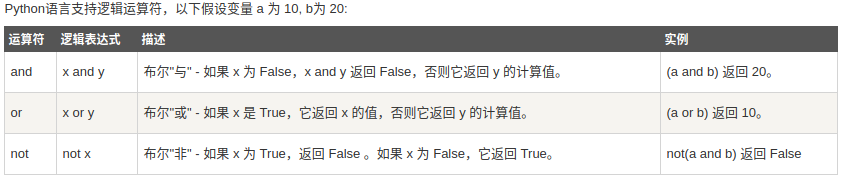实例：
#!/usr/bin/python3

a = 10
b = 20

if ( a and b ):
print ("1 - 变量 a 和 b 都为 true")
else:
print ("1 - 变量 a 和 b 有一个不为 true")

if ( a or b ):
print ("2 - 变量 a 和 b 都为 true，或其中一个变量为 true")
else:
print ("2 - 变量 a 和 b 都不为 true")

# 修改变量 a 的值
a = 0
if ( a and b ):
print ("3 - 变量 a 和 b 都为 true")
else:
print ("3 - 变量 a 和 b 有一个不为 true")

if ( a or b ):
print ("4 - 变量 a 和 b 都为 true，或其中一个变量为 true")
else:
print ("4 - 变量 a 和 b 都不为 true")

if not( a and b ):
print ("5 - 变量 a 和 b 都为 false，或其中一个变量为 false")
else:
print ("5 - 变量 a 和 b 都为 true")

输出结果：
1 - 变量 a 和 b 都为 true
2 - 变量 a 和 b 都为 true，或其中一个变量为 true
3 - 变量 a 和 b 有一个不为 true
4 - 变量 a 和 b 都为 true，或其中一个变量为 true
5 - 变量 a 和 b 都为 false，或其中一个变量为 false

Python成员运算符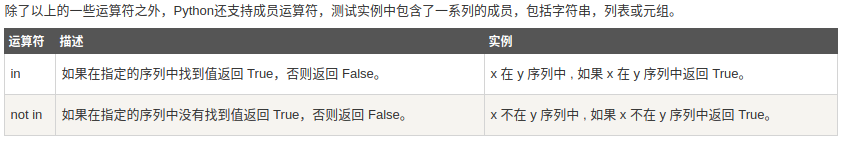实例：
#!/usr/bin/python3

a = 10
b = 20
list = [1, 2, 3, 4, 5 ];

if ( a in list ):
print ("1 - 变量 a 在给定的列表中 list 中")
else:
print ("1 - 变量 a 不在给定的列表中 list 中")

if ( b not in list ):
print ("2 - 变量 b 不在给定的列表中 list 中")
else:
print ("2 - 变量 b 在给定的列表中 list 中")

# 修改变量 a 的值
a = 2
if ( a in list ):
print ("3 - 变量 a 在给定的列表中 list 中")
else:
print ("3 - 变量 a 不在给定的列表中 list 中")

输出结果：
1 - 变量 a 不在给定的列表中 list 中
2 - 变量 b 不在给定的列表中 list 中
3 - 变量 a 在给定的列表中 list 中

Python身份运算符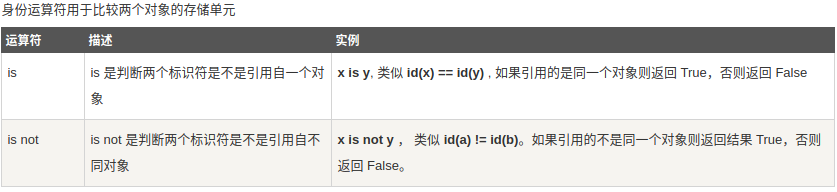实例：
#!/usr/bin/python3

a = 20
b = 20

if ( a is b ):
print ("1 - a 和 b 有相同的标识")
else:
print ("1 - a 和 b 没有相同的标识")

if ( id(a) == id(b) ):
print ("2 - a 和 b 有相同的标识")
else:
print ("2 - a 和 b 没有相同的标识")

# 修改变量 b 的值
b = 30
if ( a is b ):
print ("3 - a 和 b 有相同的标识")
else:
print ("3 - a 和 b 没有相同的标识")

if ( a is not b ):
print ("4 - a 和 b 没有相同的标识")
else:
print ("4 - a 和 b 有相同的标识")

输出结果：
1 - a 和 b 有相同的标识
2 - a 和 b 有相同的标识
3 - a 和 b 没有相同的标识
4 - a 和 b 没有相同的标识

is 与 == 区别：
is 用于判断两个变量引用对象是否为同一个， == 用于判断引用变量的值是否相等。

>>>a = [1, 2, 3]
>>> b = a
>>> b is a
True
>>> b == a
True
>>> b = a[:]
>>> b is a
False
>>> b == a
True

Python运算符优先级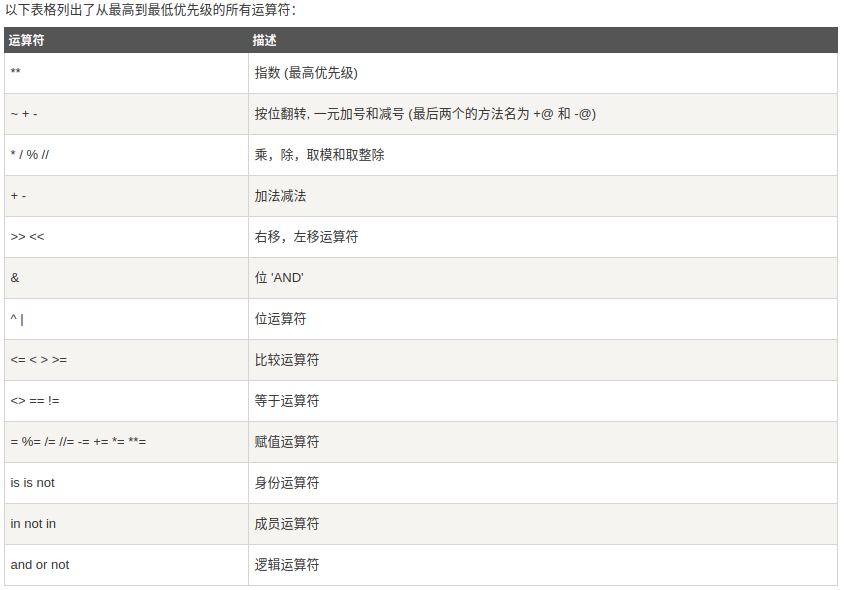实例：
#!/usr/bin/python3

a = 20
b = 10
c = 15
d = 5
e = 0

e = (a + b) * c / d       #( 30 * 15 ) / 5
print ("(a + b) * c / d 运算结果为：",  e)

e = ((a + b) * c) / d     # (30 * 15 ) / 5
print ("((a + b) * c) / d 运算结果为：",  e)

e = (a + b) * (c / d);    # (30) * (15/5)
print ("(a + b) * (c / d) 运算结果为：",  e)

e = a + (b * c) / d;      #  20 + (150/5)
print ("a + (b * c) / d 运算结果为：",  e)

输出结果
(a + b) * c / d 运算结果为： 90.0
((a + b) * c) / d 运算结果为： 90.0
(a + b) * (c / d) 运算结果为： 90.0
a + (b * c) / d 运算结果为： 50.0



展开全文• python的运算符和其他语言类似 （我们暂时只了解这些运算符的基本用法，方便我们展开后面的内容，高级应用暂时不介绍） 1.数学运算 >>>print 1+9 # 加法 >>>print 1.3-4 # 减法 >>&...
• #一、python运算符 #1.算数运算符：+，-，*，/，% #(1).+如果两边是数字，则会进行相加计算，如果两边是字符串，则会进行字符串拼接 a=2+3 print(a)#5 b="hello"+'word' print(b)#hellowordq3eeeee4...
• 整数，用int表示，来自单词：integer浮点数，用float表示，就是单词：float可以用一个命令：type(object)来检测一个数是什么类型。&gt;&gt;&gt; type(4) &lt;type 'int'&...type(988776...
• 什么是运算符？ 本章节主要说明Python运算符。举个简单的例子 4 +5 = 9 。 例子中，4 和 5 被称为操作数，"+" 称为运算符Python语言支持以下类型的运算符: 算术运算符 比较（关系）运算符 ...
• Python语言支持以下类型的运算符:算术运算符比较（关系）运算符赋值运算符逻辑运算符运算符成员运算符身份运算符运算符优先级接下来让我们一个个来学习Python运算符Python算术运算符以下假设变量a为10，变量b...
• 算术运算符：以下假设变量a为10，变量b为21：#!/user/bin/python3 a = 21 b = 10 c = 0 c = a + b print("1 - c 的值为：", c) c = a - b print("2 - c 的值为：", c) c = a * b print("3 ...
• 运算符优先级 优先级 运算符 描述  最高 ** 指数 (最高优先级)   ~ + - 按位翻转, 一元加号和减号 (最后两个的方法名为 +@ 和 -@) * / % // 乘，除，取模和取整除 + - 加法减法 ...优先级
• ## Python运算符练习

千次阅读 2019-07-12 15:39:37
Python运算符：算术，比较，关系，赋值， 逻辑 ，成员 ，身份，运算符优先级 算术运算符：±*/ %（取模） (ab,a是底数，b是指数) //(取整) 比较运算符：== ！= <>(a<>b ,类似于！=，)，> ,<, &...
• 此为Python 运算符、内置函数、序列的用法习题集，针对我的《Python 运算符、内置函数、序列的用法》博文给出的源码以及文档。文章仅12道题，适合小白学Python。不会改C币数量，第一次上传，应该是等审核后修改吧，...
• class OrderSet: def init(self,v): self.__data=set(v) def repr(self): return “OrderSet(%s)”%list(self.__data) def __and__(self,rhs): s=self.__data&amp;rhs.__data re...
• python语言是一门脚本语言，支持面向对象、面向过程编程，兼具编译性和解释性的动态语言，整理出学习过程中一些基本Python运算符和运算符的优先级顺序。 一、算术运算符 运算符 描述 + 加 - 两个对象相加 ...运算符优先级
• 由高到低依次为： 1. 幂运算：** 2. 正负号：+X，-X 3. 算术操作符：*，/，//，+，- 4. 比较操作符：&lt;，&lt;=，&gt;，&gt;=，==，!= 5. 逻辑运算符：not，and，or...
• 1、逻辑运算符 （1）and 逻辑表达式：x and y； 描述：布尔"与" --- 如果 x 为 False，x and y 返回 False，否则它返回 y 的计算值。 （2）or 逻辑表达式：x or y； 描述：布尔"或" --- 如果 x 是非 0（判断x是...
• 建议：使用多个运算符时，建议加上小括号，使得一看就懂，毕竟程序员工作代码不是只有自己看，别人也会看的。网时找到一个优先级表，保留下来，方便日后需要使用，不用找花时间找了。技术慢慢积累。。。 优先级从上...
• 所有的数学运算都是从左向右进行的，Python 语言中的大部分运算符也是从左向右结合的，只有单目运算符、赋值运算符和三目运算符例外，它们是从右向左结合的，也就是说，它们是从右向左运算的。 乘法和加法是两个...程序员 编程语言
• ## 浅析Python运算符重载

万次阅读 多人点赞 2016-09-20 23:14:38
Python语言中提供了运算符重载功能，这一点与C++有点类似，却又和C++有些许多的不同。Python运算符重载是通过重写一些Python内置的方法实现的。这些运算符方法都是以双下划线开头和结尾的，类似于__X__的形式，......